### IMO Shortlist 1999 problem G5

Kvaliteta:
Avg: 5,0
Težina:
Avg: 7,5
Let$ABC$ be a triangle,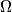$\Omega$ its incircle and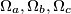$\Omega_{a}, \Omega_{b}, \Omega_{c}$ three circles orthogonal to$\Omega$ passing through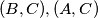$(B,C),(A,C)$ and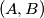$(A,B)$ respectively. The circles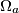$\Omega_{a}$ and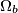$\Omega_{b}$ meet again in$C'$; in the same way we obtain the points$B'$ and$A'$. Prove that the radius of the circumcircle of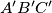$A'B'C'$ is half the radius of$\Omega$.
Izvor: Međunarodna matematička olimpijada, shortlist 1999